# MA.912.AR.4.1Export Print
Given a mathematical or real-world context, write and solve one-variable absolute value equations.
General Information
Subject Area: Mathematics (B.E.S.T.)
Strand: Algebraic Reasoning
Status: State Board Approved

## Benchmark Instructional Guide

### Terms from the K-12 Glossary

• Absolute Value

### Vertical Alignment

Previous Benchmarks

Next Benchmarks

### Purpose and Instructional Strategies

• In grade 6, students solved problems involving absolute value, including context related to distances, temperatures and finances. In grade 7, students solved multi-step order of operations with rational numbers including absolute value. In Algebra I, students write and solve absolute value equations in one variable. In later courses, students will solve and graph real-world problems that are modeled with absolute value functions.
• Instruction reinforces the definition of absolute value as a number’s distance from zero (0) on a number line. Distance is expressed as a positive value; example: |3| = 3 and |−3| = 3. The numbers 3 and –3 are each three units away from zero on a number line (MTR.2.1).
• Instruction includes asking for solutions of absolute value equation in word form. o For example, the equation |$x$| = 7.1 can be read as “What values of $x$ have absolute value equivalent to 7.1?”
• Instruction focuses on recognizing that there are either two solutions, or no solutions to an absolute value equation.
• The equation |$x$| = −8 has no solution because the absolute value of a number cannot be negative.
• The equation |5$x$ − 2| = 10 has two solutions by the definition of absolute value; one of which satisfies 5$x$ − 2 = 10 and the other satisfies 5$x$ − 2 = −10.
• Instruction encourages students to discuss their thinking with their peers (MTR.4.1). Often students will be able to reason out their thinking, but will struggle with representing their thinking mathematically. Encourage this process and ask questions that will help with solving the task (MTR.1.1). Are their multiple ways for students to represent this problem mathematically (MTR.2.1)?

### Common Misconceptions or Errors

• Students may forget that absolute value refers to a distance, and is thus a positive number or zero.
• Students may forget many absolute value equations will produce two solutions.
• Students may forget some equations have no solutions.

### Strategies to Support Tiered Instruction

• Teacher provides a three-column table with equations already sorted into two-, one-, or no-solutions. Teacher asks what is noticed about the equations in each column. Then, students sort additional equations into columns.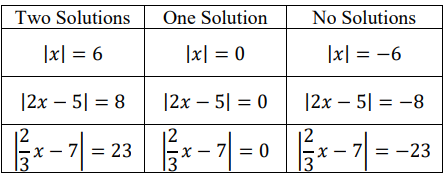• Teacher provides absolute value equations and has students them sort them into a three-column graphic organizer with column headers of: two solutions, one solution and no solution.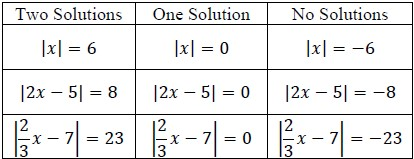• Teacher models absolute value equations on the number line to show that absolute value is the distance from zero. By the visual representation, students will see how absolute value equations produce two solutions. Manipulatives can be used to represent the constants in each equation.
• For example, the absolute value equation |$x$| = 6 can be modeled as shown.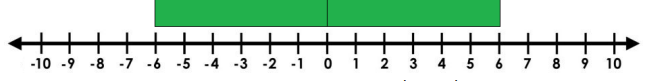• For example, the absolute value equation |$x$ + 2| = 6 can be modeled as shown.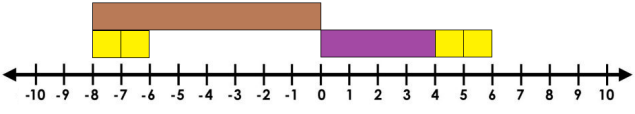• For example, the absolute value equation |2$x$ + 2| = 6 can be modeled as shown.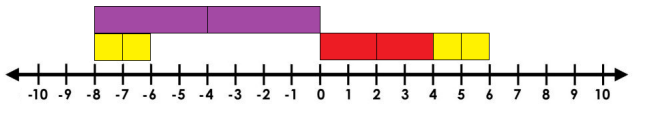• Determine the solutions of the equation below. |$\frac{\text{1}}{\text{4}}$$x$ − 3| = 10
• Determine the solutions of the equation below. |$\frac{\text{3}}{\text{2}}$$x$ + 2| + 10 = 0
• Donna and Kayleigh both go to the same high school. Donna lives 21 miles from the school. Kayleigh lives 6 miles from Donna.
• Part A. Write an absolute value equation to represent the location of Kayleigh’s house in relation to the high school.
• Part B. How far could Kayleigh live from her school?
• Jay has money in his wallet, but he doesn’t know the exact amount. When his friend asks him how much he has he says that he has 50 dollars give or take 15.
• Part A. Write an absolute value equation to model this situation.
• Part B. How much money could Jay have in his wallet?
• A car dealership is having a contest to win a new truck. In order to win a chance at the truck, you must first guess the number of keys in the jar within 5 of the actual number. The people who are within this range then get to try a key in the ignition of the truck. Suppose there are 697 keys in the jar. Write an absolute value equation that will reveal the highest and lowest guesses in order to win a chance at the truck. What are the highest and lowest guesses that will qualify for a chance to win?

### Instructional Items

Instructional Item 1
• The difference between the temperature on the first day of the month, $t$1, and the temperature on the last day of the month, 74 degrees, is 6 degrees. Write an equation involving absolute value that represents the relationship among $t$1, 74 and 6.
Instructional Item 2
• Determine the solutions of the equation below. 3|$x$ − 2| + 14 = 14

*The strategies, tasks and items included in the B1G-M are examples and should not be considered comprehensive.

## Related Courses

This benchmark is part of these courses.
1200310: Algebra 1 (Specifically in versions: 2014 - 2015, 2015 - 2022, 2022 and beyond (current))
1200320: Algebra 1 Honors (Specifically in versions: 2014 - 2015, 2015 - 2022, 2022 and beyond (current))
1200370: Algebra 1-A (Specifically in versions: 2014 - 2015, 2015 - 2022, 2022 and beyond (current))
1200400: Foundational Skills in Mathematics 9-12 (Specifically in versions: 2014 - 2015, 2015 - 2022, 2022 and beyond (current))
7912080: Access Algebra 1A (Specifically in versions: 2014 - 2015, 2015 - 2018, 2018 - 2019, 2019 - 2022, 2022 and beyond (current))
1200315: Algebra 1 for Credit Recovery (Specifically in versions: 2014 - 2015, 2015 - 2022, 2022 and beyond (current))
1200375: Algebra 1-A for Credit Recovery (Specifically in versions: 2014 - 2015, 2015 - 2022, 2022 and beyond (current))
7912075: Access Algebra 1 (Specifically in versions: 2014 - 2015, 2015 - 2018, 2018 - 2019, 2019 - 2022, 2022 and beyond (current))

## Related Access Points

Alternate version of this benchmark for students with significant cognitive disabilities.
MA.912.AR.4.AP.1: Solve a one variable absolute value equation.

## Related Resources

Vetted resources educators can use to teach the concepts and skills in this benchmark.

## Formative Assessments

Writing Absolute Value Equations:

Students are asked to solve a set of absolute value equations.

Type: Formative Assessment

Solving Absolute Value Equations:

Students are asked to solve a set of absolute value equations.

Type: Formative Assessment

## MFAS Formative Assessments

Solving Absolute Value Equations:

Students are asked to solve a set of absolute value equations.

Writing Absolute Value Equations:

Students are asked to solve a set of absolute value equations.

## Student Resources

Vetted resources students can use to learn the concepts and skills in this benchmark.

## Parent Resources

Vetted resources caregivers can use to help students learn the concepts and skills in this benchmark.